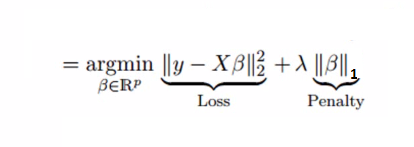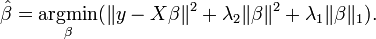# Regularization: Lasso vs Ridge

Regularization: a process of adding a tuning paramater to a model to induce the smoothness of the weights (prevent the coefficients to fit so perfectly) in order to prevent overfitting. It’s most often done by adding a constant multiple to an existing weight vector, the constant can be any norm (sometimes it will be Lasso or Ridge). Then the model predictors minimize the regularized loss function.

L1 (Lasso): the sum of the absolute value of the weights, it performs feature selection better in sparse cases• The assumptions of this regression is same as least squared regression except normality is not to be assumed
• It shrinks coefficients to zero (exactly zero), which certainly helps in feature selection.It is generally used when we have more number of features, because it automatically does feature selection.
• This is a regularization method and uses l1 regularization
• If group of predictors are highly correlated, lasso picks only one of them and shrinks the others to zero

L2 (Ridge): the sum of square of the weights, it has analytical solution, higher computational efficiency.• The assumptions of this regression is same as least squared regression except normality is not to be assumed
• It shrinks the value of coefficients but doesn’t reaches zero, which suggests no feature selection feature
• This is a regularization method and uses l2 regularization.
• It shrinks the parameters, therefore it is mostly used to prevent multicollinearity.
• It reduces the model complexity by coefficient shrinkage.

ElasticNet is hybrid of Lasso and Ridge Regression techniques. It is trained with L1 and L2 prior as regularizer. Elastic-net is useful when there are multiple features which are correlated. Lasso is likely to pick one of these at random, while elastic-net is likely to pick both.A practical advantage of trading-off between Lasso and Ridge is that, it allows Elastic-Net to inherit some of Ridge’s stability under rotation.

#### Important Points:

• It encourages group effect in case of highly correlated variables
• There are no limitations on the number of selected variables
• It can suffer with double shrinkage

L1 regularization can’t help with multicollinearity. L2 regularization can’t help with feature selection. Elastic net regression can solve both problems.